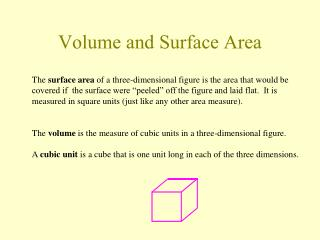DownloadDownload PresentationVolume and Surface Area

# Volume and Surface Area

Télécharger la présentation## Volume and Surface Area

- - - - - - - - - - - - - - - - - - - - - - - - - - - E N D - - - - - - - - - - - - - - - - - - - - - - - - - - -
##### Presentation Transcript

1. Volume and Surface Area The surface area of a three-dimensional figure is the area that would be covered if the surface were “peeled” off the figure and laid flat. It is measured in square units (just like any other area measure). The volume is the measure of cubic units in a three-dimensional figure. A cubic unit is a cube that is one unit long in each of the three dimensions.

2. Box The surface area of a box --- also known as a rectangular parallelepiped --- is the sum of the areas of the six sides h w l The volume of a box is the product of the three dimensions.

3. Right Circular Cylinder The surface area of a right circular cylinder --- the shape of a soup can or soda can --- is the sum of the areas of the top, the bottom and the side. The area of the side is the height of the cylinder times the distance around the cylinder. r Area of top = area of bottom= r2 Area of side = 2rh S = 2r2 + 2rh h The volume of a cylinder equals the area of the base times the height. V = r2h

4. Right Circular Cone The surface area of a right circular cone --- the shape of a witch’s hat or an ice cream cone --- is the sum of the areas of the base of the cone and the side of the cone. Area of base = r2 Area of side = r√r2 + h2 S = r2 + r√r2 + h2 h r The volume of a right circular cone is 1/3 times the area of the base times the height. V = 1/3r2h

5. Sphere To picture the surface area of a sphere imagine peeling an orange and flattening the peel on a table. The area covered would be the surface area of that sphere. S = 4r2 Note this is the area of 4 circles with radius r. r The formula for the volume of a sphere is V = 4/3r3

6. Pyramids A pyramid is a three-dimensional figure with a polygon as its base and isosceles triangles as its sides. For any pyramid if B represents the area of its base and h the height of the pyramid its volume equals 1/3 times the area of the base times the height. V = 1/3Bh h rectangular pyramid pentagonal pyramid

7. Application A rectangular office building measures 57 yds. by 36 yds. and is 28 stories high. If there are 3.5 yds. per story what is the volume of the building?

8. Application The materiel to make an aluminum soda can costs \$0.01 per square inch. How much does it cost to make a soda can 5 in. high and 2.5 in. in diameter? 2.5 in. Sweetsie Cola What is the volume of the can? 5 in.

9. Application You work at a local ice cream parlor making waffle cones. If a finished cone is 6 in. high and has a base diameter of 4 in. what is the surface area of the cone (not including the area of the base)? If you fill the cone with soft ice cream topped with a hemisphere of soft ice cream what is the volume of the ice cream?

10. Application The Transamerica Tower in San Francisco is a pyramid with a square base. Each side of the base is 52 meters and the height is 260 meters. What is the volume of the Transamerica Tower?

11. Polyhedrons A polyhedron is a 3-dimensional figure whose sides or faces are polygons. Regular polyhedrons have faces which are all the same regular polygon all equilateral triangles all squares all regular pentagons There are only 5 known regular polyhedrons: Tetrahedron --- 4 sides --- all equilateral triangles Hexahedron --- 6 sides --- all squares Octahedron --- 8 sides --- all equilateral triangles Dodecahedron --- 12 sides --- all regular pentagons Icosahedron --- 20 sides --- all equilateral triangles

12. Prisms A prism is a three-dimensional figure that has two of its faces in parallel planes These faces are congruent polygons. The rest of its faces are parallelograms formed by connecting (drawing a line between) corresponding vertices of the two congruent polygons. triangular prism trapezoidal prism